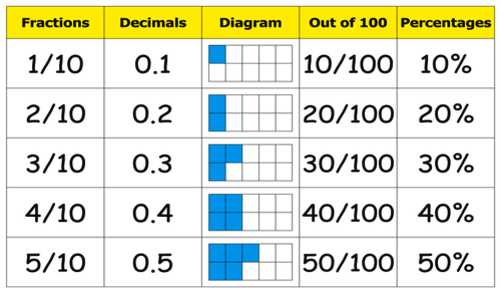Relating Equivalent Decimals to Fractions

Decimals are a type of fractional number. The decimal 0.5 represents the fraction 5/10. The decimal 0.25 represents the fraction 25/100. Decimal fractions always have a denominator based on a power of 10.

We know that 5/10 is equivalent to 1/2 since 1/2 times 5/5 is 5/10. Therefore, the decimal 0.5 is equivalent to 1/2 or 2/4, etc.

Solution process of Decimal to a Fraction follow these steps:

Step 1: Write down the decimal divided by 1, like this: decimal/1

Step 2: Multiply both top and bottom by 10 for every number after the decimal point. (For example, if there are two numbers after the decimal point, then use 100, if there are three then use 1000, etc.)

Step 3: Simplify (or reduce) the fraction

Some common Equivalent Decimals and Fractions:

• 1 and 1/10
• 2 and 1/5
• 5 and ½
• 25 and ¼
• 50 and ½
• 75 and ¾
• 0 and 1/1 or 2/2 or 1How to Convert a Decimal to a Fraction

Step 1: Make a fraction of the decimal number as the numerator (top number) and a 1 as the denominator (bottom number).

Step 2: Remove the decimal places by multiplication. First, count how many places are to the right of the decimal. Next, given that you have x decimal places, multiply numerator and denominator by 10x.

Step 3: Reduce the fraction. Find the Greatest Common Factor (GCF) of the numerator and denominator and divide both numerator and denominator by the GCF.

Step 4: Simplify the remaining fraction to a mixed number fraction if possible.

Convert a Repeating Decimal to a Fraction

• Create an equation such that x equals the decimal number.
• Count the number of decimal places, y. Create a second equation multiplying both sides of the first equation by 10y.
• Subtract the second equation from the first equation.
• Solve for x
• Reduce the fraction.

Information Source;